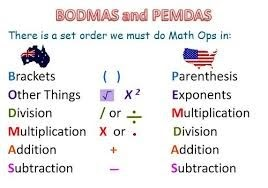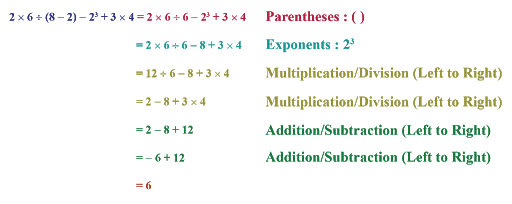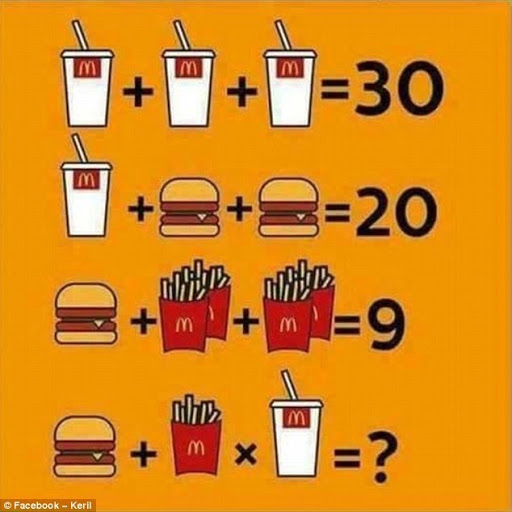Math Concepts

# PEMDAS / BODMAS

0

 1 Introduction 2 Usage of PEMDAS/BODMAS 3 When to use PEMDAS? 4 Order of Operations 5 Conditions and Rules 6 Synopsis 7 PEMDAS Quiz 8 FAQs

25th September 2020

## Introduction

Mnemonics are often needed to facilitate students to bear in mind the principles involving the first letters of words representing numerous operations. Different methods are in use in various countries.
In U. S., the shape of PEMDAS is an acronym. It stands for Parentheses, Exponents, Multiplication/Division, Addition/Subtraction. PEMDAS expanded to the mnemotechnical Please Excuse My Dear Aunt Sally.Few parts of North America and New Zealand use BEDMAS (Brackets, Exponents, Division/Multiplication, Addition/Subtraction).
Most common within the dominion, Pakistan, India, Bangladesh, and Australia and a couple of different English-speaking countries and West African countries use BODMAS (Brackets, Order, Division / Multiplication, Addition / Subtraction).

The UK uses BIDMAS (Brackets, Indices, Division / Multiplication, Addition / Subtraction).## Usage of PEMDAS / BODMAS

The order of operations that employed throughout arithmetic, science, technology, and many of programming language expressed here:

1.  Exponentiation and Root Extraction
2.  Multiplication and Division

It means that if during a mathematical expression, a subexpression seems between 2 arithmetic operators, the operator that is higher within the upper list needs to be applied initially.

Here is an example of PEMDAS.## When to use PEMDAS?

• When there is more than one arithmetic operation in a mathematical expression, we use the PEMDAS rule.
• PEMDAS in mathematics provides you with a correct structure and unique declaration of each mathematical expression.
• There is a sequence of a few rules that are required to follow once victimization the PEMDAS methodology.
• Once you get the suspension of those rules, you will be able to do multiple steps quickly.

Important notes to recollect:

• Operations in brackets ought to administer initially.
• Next, solve the exponents within the expression.
• Move from left to right and do Multiplication or division, whichever comes first.
• Move from left to right and do addition or subtraction, whichever comes first.

## Order of Operations

Do things in Brackets initially.

 4 × (5 + 3) = 4 × 8 = 32 4 × (5 + 3) = 20 + 3 = 23 (Wrong)

Exponents (Powers, Roots) before Multiply, Divide, Add, or Take away.

 5 × 22 = 5 × 4 = 20 5 × 22 = 102 = 100 (Wrong)

Multiply or Divide before you Add or Take away.

 2 + 5 × 3 = 2 + 15 = 17 2 + 5 × 3 = 7 × 3 = 21 (Wrong)

Otherwise, go from left to right.

 30 ÷ 5 × 3 = 6 × 3 = 18 30 ÷ 5 × 3 = 30 ÷ 15 = 2 (Wrong)

How Do I remember it All ...? BODMAS!

 B Brackets first O Orders (i.e., Powers and Square Roots, etc.) DM Division and Multiplication (left-to-right) AS Addition and Subtraction (left-to-right)

• Divide and Product rank equally (and go left to right).
• Add and Take away rank equally (and go left to right).

So, take it this way:

• After you have got B and O, go from left to right doing any D or M as you discover them.
• Then go from left to right doing any A or S as you see them.

## Conditions and Rules PEMDAS / BODMAS

These conditions and rules for the general simplification of expression below:

Condition Rule
a+ (b + c) ⇒ a + b + c  Open the bracket and sum the terms.
a– (b + c) ⇒ a–b–c Open the bracket and multiply the negative sign with every term inside them. (All Positive terms are negative and vice-versa)
a (b + c) ⇒ ab + ac  Multiply the outside term with every term within the bracket

300 - (4 x 2² + 12)

We have to look at the parentheses 1st, remember. Three arithmetic operators within these brackets that tend to use PEMDAS to work out that we would like to tackle the exponent 1st.

300 - (4 x 4 + 12)

Still performing on the parentheses, there is a multiplication that ranks next.

300 - (16 + 12)

Let us end the parentheses.

300 - 28

And the final operation is that the minus.

300 - (4 x 2² + 12) = 272

### How Did You Do?

Hopefully, you managed to induce all the answers right. If not, return and review wherever you went wrong, and skim over the principles another time.

The more you practice, the simpler PEMDAS / BODMAS becomes, and eventually, it will not even get to consider it.

As of recently, riddles like this,appeared where one overlooks that the number of fries has modified. Or this:These kinds of puzzles have managed to become viral, not due to the PEMDAS factor as a result of individuals who do not scrutinize the variations (Three rather than four bananas, 2 o’clock instead of 3 o’clock). Nearly everyone gets it wrong initially. However, there is additionally the PEMDAS challenge. Some get 88. except for obtaining 88, one would get to write parenthesis (2+3+3) *11.

Try these calculations:

•  3 + 20 × 3 =?
•  25 - 5 ÷ (3 + 2) =?
•  10 + 6 × (1 + 10) =?
•  5 (3 + 2) + 52 =?
•  (100 + 211) - 550 ÷ 52 + 10 =?

## Synopsis

I hope this text has helped you to urge you to grips with what BODMAS, BIDMAS, and PEMDAS area unit. For additional in-depth scrutiny of the Order of Operations, at the side of some attention-grabbing examples, you may adore it. However, do not expect to flee while not a headache.

TIP: Work it out piecemeal and do not attempt to take shortcuts.

## PEMDAS Quiz

Think you recognize your PEMDAS from your elbow? Try our quiz to ascertain if you have it nailed! There is no prize, aside from the bragging rights of being prime of the class!

## 1. What is the rule for BODMAS?

The BODMAS rule gives us the correct sequence for solving a mathematical expression.
In BODMAS rule, operations are performed in parentheses first.
Next, operations are performed on exponents or powers.
This is followed by the operations on multiplication or division from left to right, whichever comes first.
Finally, the operations on addition or subtraction are performed from left to right, whichever comes first.

## 2. Do you multiply or divide first in BODMAS?

In BODMAS rule, we solve operations on multiplication and division from left to right.
We perform the operation that comes first.

## 3. When do we apply the BODMAS rule?

BODMAS Rule is applied for solving difficult mathematical expression involving more than one operation like addition, subtraction, multiplication or division.

-Written by Priti Jain, Cuemath Teacher

Related Articles
GIVE YOUR CHILD THE CUEMATH EDGE
Access Personalised Math learning through interactive worksheets, gamified concepts and grade-wise courses